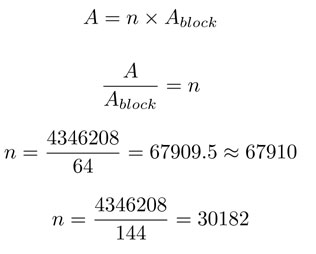SEARCH HOMEMath Central Quandaries & QueriesQuestion from Simone: Hi there, I have a quote to complete and I need to figure out the formula that will tell me how many concrete blocks I need to cover a certain area. Blocks can either be 8in or 12in. The area that I am looking to cover with blocks is 30182 sq ft. Thank you for your assistance.We have two responses for you

Hi Simone,

To determine how many blocks are needed, you need to divide the total area to be covered by the area a single block. I assume you mean that the blocks have a length and width of 8 in × 8in and 12 in × 12 in which result in areas of 64 sq inches and 144 sq inches. We need to have the same units for both the area to be covered and area of the block so I converted 30182 sq ft into 4346208 sq inches. For the following equation A is the area to be covered, Ablock is the area of a single block and n is the number of blocks needed:We previously answered a similar question concerning how many cards can be fit on a round table.

Hope this helps,

Janice

Hi Simone. I'm not all that conversant with American construction materials, so I'm assuming you mean blocks like those described here: http://www.westbrookblock.com/products/12inch.htm

Such blocks are 8 in x 16 in and the depth can be 8 inches or 12 inches (or a few other options). I'm not sure how you are placing them either, so I will take a guess and say that it is the 8 x 16 side that is "exposed" and covering the area you gave me.

This means that I am presuming that an 8 inch block and a 12 inch block cover the same area, but differ in terms of thickness only. If this is not your situation, you will simply have to provide more information to us. We are mathematicians after all, not construction experts.

It's my understanding that concrete blocks are slightly smaller than advertised in order to allow for the mortar spacing, so I can just ignore the mortar area.

Thus, each installed block covers 8 in x 16 in of surface. That's 128 square inches of surface.

Now let's compare that to 30 182 sq feet. Each foot is 12 inches. So each square foot is 12 squared inches squared. That means 144 square inches per square foot. 30 182 x 144 equals 4 346 208 square inches.

Now we simply divide this total area by the area each block covers:

4 346 208 / 128 = 33 955. That's just under thirty four thousand blocks.

Cheers,
Stephen La Rocque.Math Central is supported by the University of Regina and The Pacific Institute for the Mathematical Sciences.